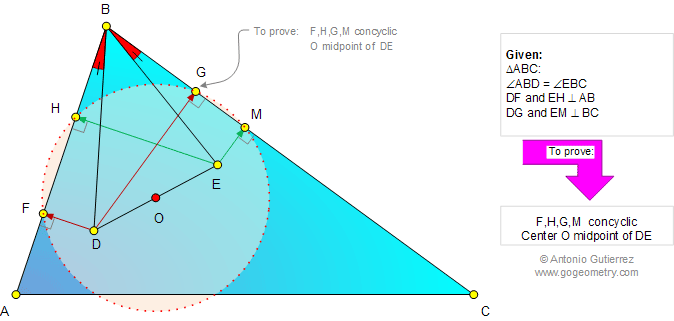# Online Geometry Problem 776: Triangle, Isogonal lines, Congruent angles, Perpendicular, Concyclic points, Circle, Center, Midpoint. Level: High School, College, Mathematics Education

 The figure below shows a triangle ABC with angle ABD = angle EBC (isogonal lines). DF and EH are perpendicular to AB, DG and EM are perpendicular to BC. Prove that F, H, G, M lie on a circle (concyclic points) whose center O is the midpoint of DE.Home | Search | Geometry | Problems | All Problems | Open Problems | Visual Index | 771-780 | Triangles | Isogonal Lines | Angles | Perpendicular lines | Circles | Cyclic Quadrilateral | Concyclic Points | Midpoint | Email | Solution / comment | By Antonio Gutierrez